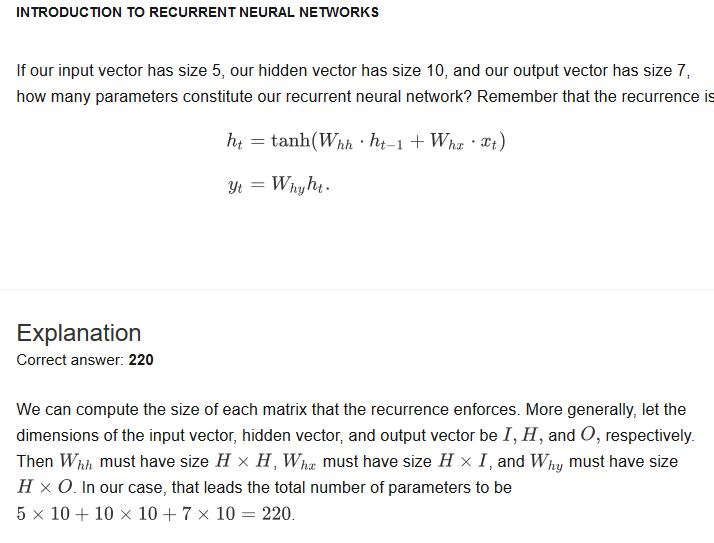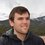# Recurrent Neural Network Hidden Vector

How is it possible to add two vectors of different sizes for the hidden vector formula? Simple vector/matrix addition is defined only for vectors/matrices of the same size. If the hidden vector and input vector are different sizes (ie. number of rows for the column vectors), then how is addition defined between them?

I am having difficulty understanding the following problem in the Artificial Neural Networks course (Recurrent Neural Networks Quiz 1 Problem 10).

The problem is the following:

"If our input vector has size 5, our hidden vector has size 10, and our output vector has size 7, how many parameters constitute our recurrent neural network? Remember that the recurrence isNote by Matt Bryk
2 years, 8 months ago

This discussion board is a place to discuss our Daily Challenges and the math and science related to those challenges. Explanations are more than just a solution — they should explain the steps and thinking strategies that you used to obtain the solution. Comments should further the discussion of math and science.

When posting on Brilliant:

• Use the emojis to react to an explanation, whether you're congratulating a job well done , or just really confused .
• Ask specific questions about the challenge or the steps in somebody's explanation. Well-posed questions can add a lot to the discussion, but posting "I don't understand!" doesn't help anyone.
• Try to contribute something new to the discussion, whether it is an extension, generalization or other idea related to the challenge.

MarkdownAppears as
*italics* or _italics_ italics
**bold** or __bold__ bold
- bulleted- list
• bulleted
• list
1. numbered2. list
1. numbered
2. list
Note: you must add a full line of space before and after lists for them to show up correctly
paragraph 1paragraph 2

paragraph 1

paragraph 2

[example link](https://brilliant.org)example link
> This is a quote
This is a quote
    # I indented these lines
# 4 spaces, and now they show
# up as a code block.

print "hello world"
# I indented these lines
# 4 spaces, and now they show
# up as a code block.

print "hello world"
MathAppears as
Remember to wrap math in $$ ... $$ or $ ... $ to ensure proper formatting.
2 \times 3 $2 \times 3$
2^{34} $2^{34}$
a_{i-1} $a_{i-1}$
\frac{2}{3} $\frac{2}{3}$
\sqrt{2} $\sqrt{2}$
\sum_{i=1}^3 $\sum_{i=1}^3$
\sin \theta $\sin \theta$
\boxed{123} $\boxed{123}$

Sort by:

Hi Matt;

Thank you for asking this question.

You are correct to note that vectors cannot be added unless their dimensions match. This is the reason why we need to look at the transformation $W_{hx}$. In other words, this matrix should be such that it can modify the input vector from size 5 to size 10 with some suitable transform.

- 2 years, 8 months ago

Thank you for the response Agnishom. In other words, we choose the number of rows and columns, for Whx and Whh, such that the products of matrices (Whx)(Xt) and (Whh)(Ht-1) have the same dimensions? So that way we can now sum the products (Whx)(Xt) and (Whh)(ht-1).

Are the dimensions of the input vector and hidden vector allowed to change through time? For example, can ht have different dimensions than ht-1? Alternatively, can we have xt with different dimensions than xt-1? If yes, how do we construct Whx and Whh with changing input and hidden vector dimensions?

- 2 years, 8 months ago

Hi Matt, Your first statement looks correct, the recurrence relation works because we pick out our matrices to make sure all products match.

Changing our dimensions with time is a very interesting thought, but in our case, because of how we've defined recurrent networks, it is not possible. The dimensions of our vectors can't change with time because the transformation matrices in our recurrence relation are constant throughout all time steps. They can only ever take in the same number of inputs and outputs, so there's no way to increase or decrease the dimensions of our hidden or input vectors.

Theoretically, you could define many transformation matrices, or come up with some novel way of handling the problem, but this isn't an approach that's been studied, and the architecture would no longer be a normal recurrent network. We can't say much about how you'd go about constructing this, or what its properties would be.

Staff - 2 years, 8 months ago